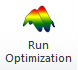Include Top

# Overview of DiscoverSim™ Menu and Dialogs

## Run Optimization1. Optimization Goal/Multiple Output Metric: Minimize or Maximize Weighted Sum: minimize or maximize the weighted sum of statistic. Weights are specified for each output in the Output Response dialog. For example, if the selected statistic was the Mean, and there were two outputs, the objective function would be:

• Weight1Mean1 + Weight2Mean2

Note, if there is a single output in the model, this simplifies to minimize or maximize the statistic value.

Note that the Output Goal specified in the Output Response dialog is not used here. It is only used in Maximize Desirability.

2. Optimization Goal/Multiple Output Metric: Minimize Deviation from Target: minimize the square root of weighted sum of deviations squared. A target must be specified for each output in the Output Response dialog. The only statistic available for this option is the mean. This is also known as the Taguchi or Quadratic Loss Function. If there were two outputs, the objective function would be:

• SQRT(Weight1(Mean1 – Target1)2 + Weight2(Mean2 – Target2)2)

3. Optimization Goal/Multiple Output Metric: Maximize the weighted linear sum of the Desirability Function (Derringer and Suich, 1980). Each output must specify:

• •  Weight (default = 1). This is also referred to as “Importance”. (Note, another factor, the desirability shape is sometimes called “weight”. In DiscoverSim, the desirability shape factor is fixed at 1.)

• •  Output Goal (Target, Maximize or Minimize) – this is specific to an output. For example, if Output 1 is production rate, the goal would be set to maximize, and Output 2, cost, would have a minimize goal. However the specified overall objective function goal is to maximize desirability.

• If the output goal is Target, then LSL, Target, and USL are required. LSL and USL are the lower and upper specification limits used for process capability and dpm calculations, but are also used as the lower and upper bounds for desirability.

• If the output goal is Minimize, then Target and USL are required.

• If the output goal is Maximize, then LSL and Target are required.

The only statistic available for this option is the mean.

4. For further details on Multiple Output Metrics see the Appendix: Formulas for Multiple Output Metrics.

5. Statistic: The following tables give the Statistic options available depending on the selected Optimization Goal and Multiple Output Metric:

6.  Optimization Goal: Minimize Maximize Multiple Output Metric: Weighted Sum Deviation from Target Weighted Sum Desirability Statistic: MeanMedian1st quartile3rd quartilePercentile (%)MinimumMaximumStandard DeviationVarianceMean Squared Error (requires Target)SkewnessKurtosisRangeIQR (75-25)Span (95-5)Actual DPM (defects per million - requires LSL/USL)Actual DPMU (Upper – requires USL)Actual DPML (Lower – requires LSL)Calculated DPM (defects per million assuming normal distribution - requires LSL/USL)Calculated DPMU (Upper – requires USL)Calculated DPML (Lower – requires LSL) Mean (requires Target) MeanMedian1st quartile3rd quartilePercentile (%)MinimumMaximumStandard DeviationVarianceMean Squared Error (requires Target)SkewnessKurtosisRangeIQR (75-25)Span (95-5)Pp (requires LSL/USL)PpU (Upper – requires USL) PpL (Lower – requires LSL)Ppk (requires LSL/USL)Cpm (requires Target/LSL/USL)%Pp (Percentile Pp – requires LSL/USL)%PpU (Percentile PpU – requires USL)%PpL (Percentile PpL – requires LSL)%Ppk (Percentile Ppk – requires LSL/USL) Mean (requires Target and LSL, USL or LSL/USL)

7. Formulas for general statistical measures are given in the DiscoverSim Workbook Appendix: Formulas for Statistical Measures.

8. Formulas for Quality and Process Capability Indices are given in the DiscoverSim Workbook Appendix: Formulas for Quality and Process Capability Indices.

9. DiscoverSim includes the following Global and Local optimization methods:

• •  Mixed Integer Distributed Ant Colony Global Optimization (MIDACO)

• •  Genetic Algorithm (GA) Global Optimization

• •  Discrete Exhaustive optimization – all combinations for small discrete problems

• •  Sequential Quadratic Programming (SQP) for fast local smooth optimization

• •  Nelder-Mead (NM) simplex optimization for fast local non-smooth problems

• •  Powerful Hybrid of the above methods:

• All discrete controls: MIDACO or Exhaustive Discrete if applicable

• All continuous controls: MIDACO, GA, followed by SQP or NM

• Mixed continuous/discrete controls: MIDACO 1 (Course), MIDACO 2 (Fine), followed by SQP or NM

• See the DiscoverSim Workbook Appendix: HYBRID Optimization for more details, including Advanced Settings for Hybrid.

• •  Note that the Advanced Settings button will open a dialog with settings specific to the selected method.

10. Replications value sets the number of replications used in optimization to obtain the Statistic. The default value is 1000.

11. Seed is set to Clock by default so that the starting seed of random number generation will be different with each run. If you want the optimization results to match every time (for example in a classroom setting where you want all students to obtain the same results), select Value and enter an integer number. Stochastic optimization requires a fixed seed in order to avoid “chatter” that would result in inconsistent comparisons. If the Seed is set to Clock, the initial seed value will be obtained from the system clock and then kept fixed throughout the optimization. Note that the results of a fixed seed for 32-bit Excel will be slightly different for 64-bit Excel.

12. Select Monte Carlo (Random) for full randomization. Latin Hypercube Sampling is less random than Monte Carlo but enables more accurate simulations with fewer replications.

13. Accelerated Mode uses DiscoverSim’s Excel Formula Interpreter to dramatically increase the speed of calculations for rapid optimization. If unchecked, the calculations are performed using native Excel. The interpreter supports the majority of all Excel numeric functions (for more details see the DiscoverSim Workbook Appendix: DiscoverSim Engine and Excel Formula Interpreter). If the DiscoverSim interpreter sees a function that it does not support, you will be prompted to use the Excel Native mode.

14. Check Independence (Ignore Correlations) to run the optimization with all inputs independent of each other (zero correlation).

15. Optimization in process may be interrupted/paused or stopped. Upon completion, the user can paste the optimum input control values in order to perform further simulation studies.

# Web Demos

Our CTO and Co-Founder, John Noguera, regularly hosts free Web Demos featuring SigmaXL and DiscoverSim